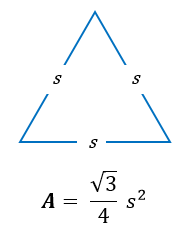# Basic Java programming exercises

 Java Tutorial Java Exercises Data Structures

## Java program to find area of an equilateral triangle

Write a Java program to input side of an equilateral triangle from user and find area of the given triangle. How to find area of an equilateral triangle in Java programming. Java program to calculate area of an equilateral triangle if its side is given.

##### Required knowledge

Arithmetic operators, Variables and expressions, Basic input/output

##### Area of an equilateral triangle

Area of an equilateral triangle is given by formula.Java equivalent expression to find area of equilateral triangle - (sqrt(3) / 4) * (side * side)

##### Logic to find area of equilateral triangle

Mathematical formula for area of equilateral triangle in programming notation can be written as (sqrt(3) / 4) * (side * side). Where sqrt() is a function used to compute square root. We will use this formula to find area of equilateral triangle.

Below is the step by step descriptive logic to find area of an equilateral triangle.

1. Input side of the equilateral triangle. Store it in some variable say side.

2. Use the formula of equilateral triangle to find area i.e. area = (sqrt(3) / 4) * (side * side).

3. Finally, print the value of resultant area.

##### Program to find area of an equilateral triangle
```/**
* Java program to find area of an equilateral triangle
*/

import java.util.Scanner;

class Test
{
public static void main(String args[])
{
double side, area;
Scanner op=new Scanner(System.in);
/* Input side of equilateral triangle */
System.out.print("Enter side of an equilateral triangle: ");
side=op.nextDouble();

/* Calculate area of equilateral triangle */
area = (Math.sqrt(3) / 4) * (side * side);

/* Print resultant area */
System.out.println("Area of equilateral triangle = "+area+" sq. units");
}
}
```

##### Output:

```Enter side of an equilateral triangle: 5
Area of equilateral triangle = 10.825317547305483 sq. units
```